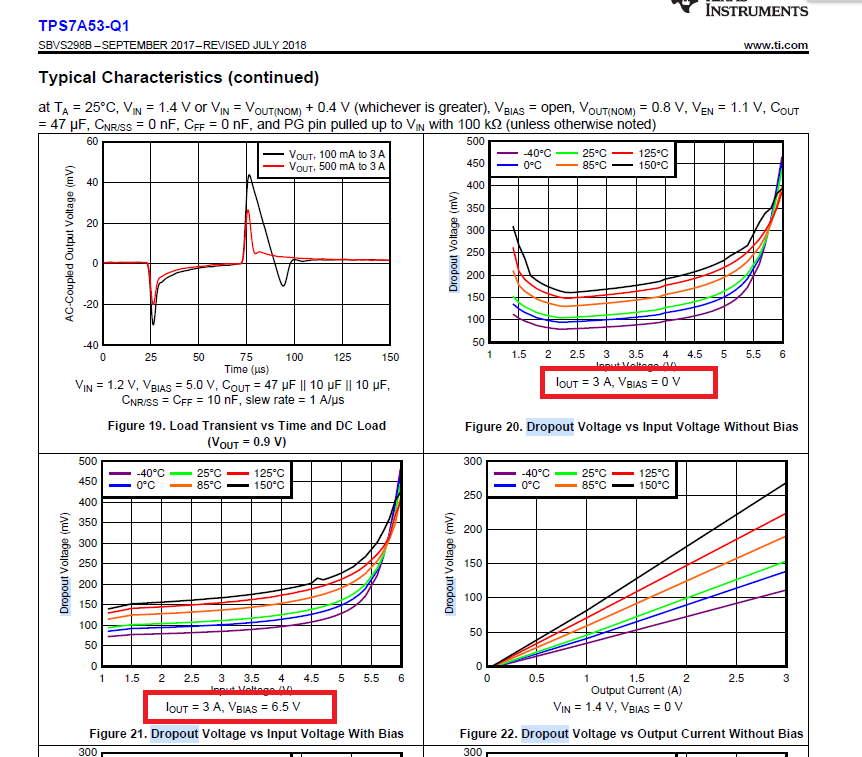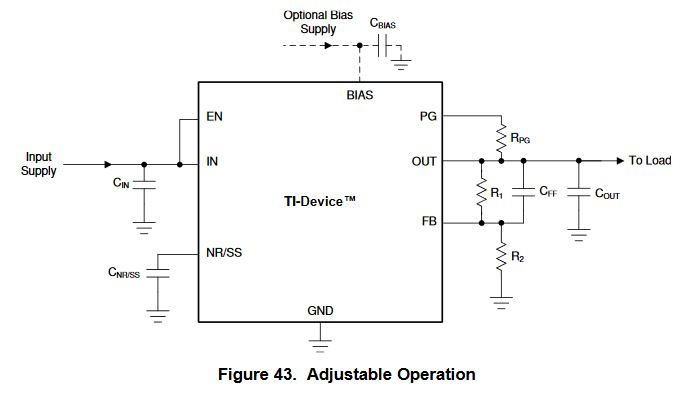If you have a related question, please click the "Ask a related question" button in the top right corner. The newly created question will be automatically linked to this question.

TPS7A53-Q1: Low VIN application issue when designing tps7a53

Part Number: TPS7A53-Q1
Other Parts Discussed in Thread: TPS7A53,

Hi, team,

now i have one question on TPS7a53 for low VIN input application, could you please help provide the total solution for it? thanks.

application request: Vin = ~1.4V,  Vout = 1.15V (exactly), Iout <=2.5A, automotive EE.

chip: TPS7a53-q1 is one candidate

question: from d/s, the dropout voltage is <=320mV @3A with Vias =0. from the picture shown below, when Vbias is used, the headroom could be much smaller (indicated in figure21). this is helpful for me as I also consider to use Vin = 1.35V to get 1.15V output. so please help check if I can design this with Vin=1.35V and BIas voltage is set as 6.5V? If yes, if we can use the resistor divider (10K + 10K)  to generate the 6V  bias voltage? any better bias voltage is recommended here?

btw, can you help attach the total "verified solution for it? thanks.• Hi Junqiang,

At 2.5A load current or less, you will typically need 225mV of headroom or less, and this is at the highest temperature of 150C.
At 1.4V input and 1.15V output, and 2.5A max load, you have 625 mW of power being dissipated across the LDO, worst case.
The thermal resistance for the VQFN package is 43.4 C/W, which gives you a thermal increase of about 27 degrees.  So if your ambient temperature is less than 100 degrees Celsius, your max dropout will only be 175mV giving you even more margin to your 1.4V input and 1.15V output.

If you choose to use a seperate Vbias supply, I would not recommend a voltage divider using 10 k-ohm resistors.  The bias rail needs to supply some nontrivial current to operate the internal circuitry.  The datasheet lists 2.4 mA typical and 3.5 mA maximum, which would result in a significant voltage drop across the 10 k-ohm resistors.  It would be better to use a separate voltage rail that can provide the voltage.  Anything higher than Vout + dropout on the bias rail would be sufficient.

As for a starting schematic solution, I would start with the application schematic in the Application section of the datasheet.

From section 8 in the datasheet, set the following values:

Cin = 10uF or greater (5uF effective capacitance)
Cbias = 10uF or greater (5uF of effective capacitance)
Cout = 47uF or greater (22uF effective capacitance).  The usual recommendation is 47uF // 10uF // 10uF as described in the datasheet.
C_nr/ss = 10nF to 1uF
Cff = not required but can be 10nF for improved noise performance

Rpg = 10 k-ohms to 100 k-ohms

To generate 1.15V on the ouput, you can set R1 = 12.1 k-ohms and R2 = 27.4 k-ohms.Thanks,

- Stephen

• Thank you for detail, Stephen.

so in this case, as i have no dedicated bias voltage in this case, can I link Vin (~1.4V) to Bias voltage directly? from d/s, it is recommended to connect BIAS voltage when VIN is <=2.2V. If yes, if this can further improve the headroom of dropout voltage?

in conclusion, if I select Vin=1.35V,and connect Bias pin to Vin pin, if I get get stable 1.15V output when output current is among the range of 30mA to 2.5A? thanks.

best regards,

Steven